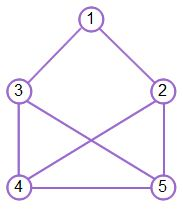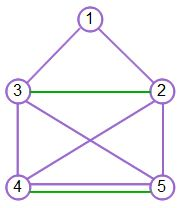Eulerizing Graphs in Math

Lesson Transcript
Instructor: Yuanxin (Amy) Yang Alcocer

Amy has a master's degree in secondary education and has been teaching math for over 9 years. Amy has worked with students at all levels from those with special needs to those that are gifted.

Eulerizing graphs in math has direct real-world applications. Examine the differences between Eulerized and semi-Eulerized graphs, and learn how to apply Fleury's Algorithm in order to create them. Updated: 10/30/2021

A Graph

We begin with a graph - this graph:Now, imagine that you are a chief engineer for the town of Harrisville. Your job is to create roads that will make it easy to navigate the town for both residents and workers, especially the mailman. You want to build the roads in such a way so that the mailman can have an optimized route where he passes each road only once.

In order to do this, you make use of your knowledge of graph theory. Why? Because this graph that you are looking at is actually a drawing of the current roads of the town. The intersections are marked with the dots with numbers in them. You know that when a graph has an Euler circuit or Euler path, then that means the graph is mailman-friendly because there is a route that he can take to pass each road just once. The only difference being that the path takes the mailman to a different end point, and the circuit has the mailman ending where he began.An error occurred trying to load this video.

Try refreshing the page, or contact customer support.

Coming up next: Hamilton Circuits and Hamilton Paths

You're on a roll. Keep up the good work!

Replay
Your next lesson will play in 10 seconds
• 0:02 A Graph
• 1:01 Fleury's Algorithm
• 1:49 Eulerizing a Graph
• 3:20 Semi-Eulerizing a Graph
• 5:05 Lesson Summary
Save Save

Want to watch this again later?

Timeline
Autoplay
Autoplay
Speed Speed

Fleury's Algorithm

You also make use of Fleury's algorithm that tells you that when a graph has zero odd vertices, then it has an Euler circuit, and when the graph has two odd vertices, then it has an Euler path. Knowing this helps you to analyze your current graph to see if the roads are already mailman-friendly. You count the number of odd vertices; remember that an odd vertex is a vertex where the number of edges connecting it to other vertices is odd. In your graph, you count four odd vertices. Hmmm. This means that there currently is no Euler path or Euler circuit in this graph. What are you to do?

Since you are the chief engineer for this town, you go ahead and propose some new roads. But where should you place these roads? Which intersections should the new roads connect?

Eulerizing a Graph

The purpose of the proposed new roads is to make the town mailman-friendly. In graph theory terms, we want to change the graph so it contains an Euler circuit. This is also referred to as Eulerizing a graph. The most mailman-friendly graph is the one with an Euler circuit since it takes the mailman back to the starting point. This means that the mailman can leave his car at one intersection, walk the route hitting all the streets just once, and end up where he began. There is no backtracking or walking of streets twice. This saves him time.

We used Fleury's algorithm to help us determine whether our graph has an Euler circuit to begin with. We can also use Fleury's algorithm to help us decide where to place our new roads, our new edges. According to Fleury's algorithm, in order for a graph to have an Euler circuit, all of the vertices must be even, meaning we have zero odd vertices. To accomplish this, we can draw new lines connecting vertices that are odd together to make them even.

For example, we can connect vertices 3 and 2 together. This changes these two vertices from odd to even. Now we are left with two odd vertices, vertices 4 and 5. To change these to even vertices, we can add another road connecting these two. This changes our graph to one where there are zero odd vertices, meaning that we have a graph with an Euler circuit in it. We have Eulerized our graph by adding two new roads.To unlock this lesson you must be a Study.com Member.

Register to view this lesson

Are you a student or a teacher?